Courses

# IIT JAM Physics MCQ Test 12

## 30 Questions MCQ Test Mock Test Series for IIT JAM Physics | IIT JAM Physics MCQ Test 12

Description
This mock test of IIT JAM Physics MCQ Test 12 for Physics helps you for every Physics entrance exam. This contains 30 Multiple Choice Questions for Physics IIT JAM Physics MCQ Test 12 (mcq) to study with solutions a complete question bank. The solved questions answers in this IIT JAM Physics MCQ Test 12 quiz give you a good mix of easy questions and tough questions. Physics students definitely take this IIT JAM Physics MCQ Test 12 exercise for a better result in the exam. You can find other IIT JAM Physics MCQ Test 12 extra questions, long questions & short questions for Physics on EduRev as well by searching above.
QUESTION: 1

### If α = 0.98. ICO = 6 μA and lR = 100μA for a transistor, then the value of lc will be

Solution: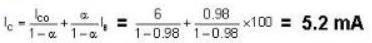QUESTION: 2

### For the given input, the output waveform across the diode shown in the figure. will be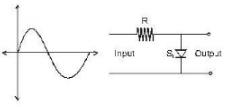Solution:

The diode will not conduct in the negative half of the wave and while it conducts, there will be a small voltage drop across, it shown as 0.06 V on option (D)

QUESTION: 3

### Which of the following option correctly represents the dependence of mobility with temperature?

Solution:

Mobility μh & μp have a temp. dependence & is approximately expressed as μ ∝ T-3/2. Opyion (D) is the correct option.

QUESTION: 4

The graph of [logρ vs. 1/T] can be expressed as:-

Solution:

As (ρ) resistivity = (constant).exp (+Eg/2KT)
Log ρ = log (constant) + Eg/2KT

QUESTION: 5

Which is correct logic diagram of the simplified form of given boolean expression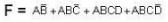Solution: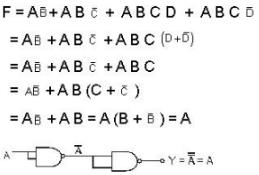QUESTION: 6

F(ABC) = (A + B + AB) (A + C + AC) has simplified expression

Solution:

F(A, BC) = [A + B(1 +A)] [A +C (1+A)]
=  (A+B) (A+C) = A + AB + BC + AC
= A(A+B) + BC+AC
= A + AC + BC
= A(1+C) + AC
= A + BC

QUESTION: 7

An OPAMP has a slew rate of 5 V/μs. The largest sinewave output voltage possible at a frequency of 1 MHz is

Solution:

V = Vm sin ωt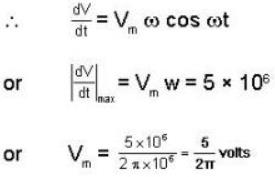QUESTION: 8

For the logic circuit shown in the figure below, the output Y is equal to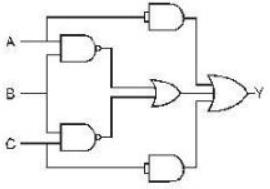Solution:QUESTION: 9

The output voltage of an OPAMP for input voltage of Vi1 = 150 μV , Vi2 = 140μV if the amplifier has a differential gain of Ad = 4000 and the value of CMRR is 100, is

Solution: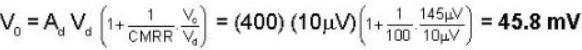QUESTION: 10

A non-inverting op-amp summer is shown in the figure. The output voltage Vo will be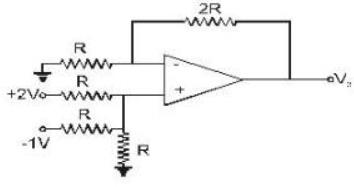Solution: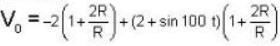= - 6 + 6 + 3 sin 100t = 3 sin 100 t

QUESTION: 11

The Q point of the transistor circuit given below is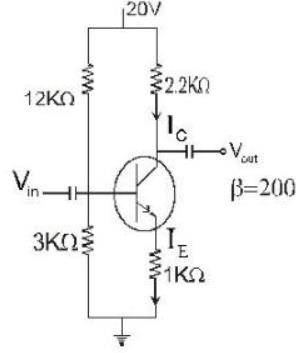Solution:

Using approximation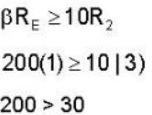So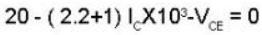...(i)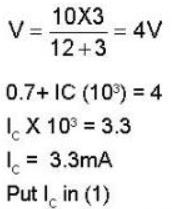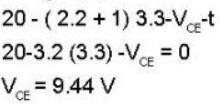QUESTION: 12

The saturation current for the given network is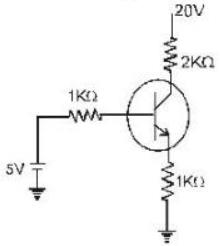Solution:

(Ic) saturation =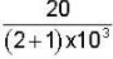20/3 mA= 6.67mA

QUESTION: 13

The value of VCE for the voltage - divider bias configuration is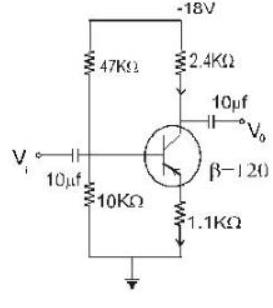Solution:

Testing the condition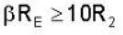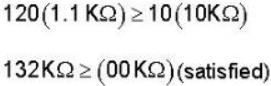Solving for VB we have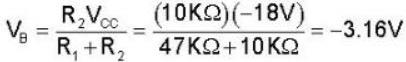Apply KVL around base emitter loop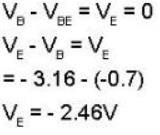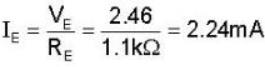For collector - emitter loop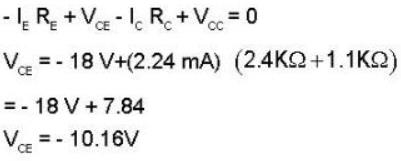QUESTION: 14

For the CB circuit shown below in figure ; The value of ‘ Ic ’ will be:-  ( Assume The transistor to be of silicon)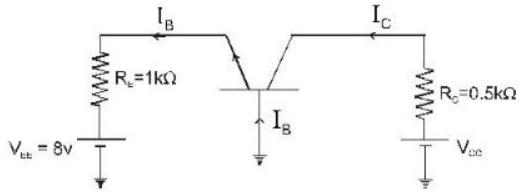Solution: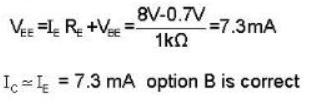QUESTION: 15

For the circuit given below; calculate the value of VCB (Assuming the transistor to be of silicon.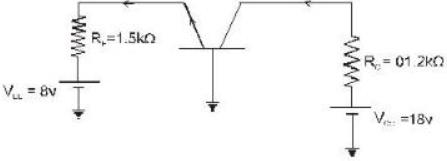Solution: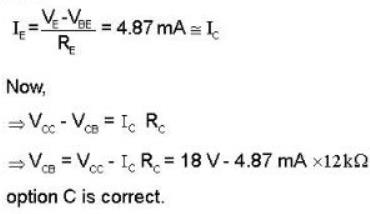*Multiple options can be correct
QUESTION: 16

Choose the correct statement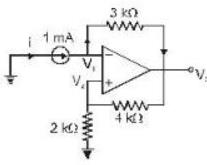Solution:

We note that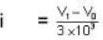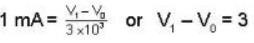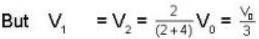so putting in eq. (1), we get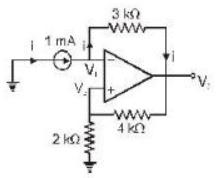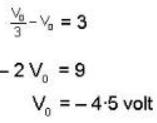and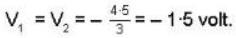*Multiple options can be correct
QUESTION: 17

For the given circuit we have R1 = 6 kΩ, Rf = 24 kΩ, Vi = 1 V and load resistor of 6 kΩ. Then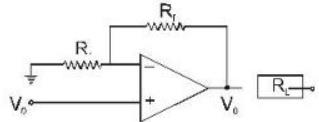Solution: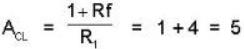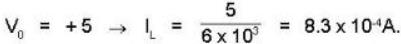*Multiple options can be correct
QUESTION: 18

Fora differential amplifier in which the signal applied to inverting and non inverting term inals are respectively = - 0.45 m V and + 0.48 m V and A diff = 106 and CMRR = 80 dB.

Solution: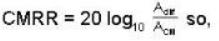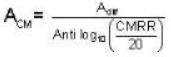So,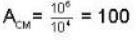The differential input is
Vdff = V2 - V1 = - 0.48 - (0.45) mV = -.03mV and common mode input is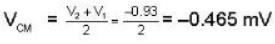Total output voltage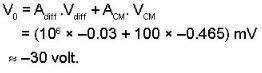*Multiple options can be correct
QUESTION: 19

In a given n-type silicon material, the donar concentration is 1 atom per 2 * 108 silicon atoms. Assuming that the effective mass of the electron is equal to its true mass. Given that density of atoms in silicon = 5 * 1028 atoms/m3. Which of the following statement is correct.

Solution:

The donor consecration will be calculated first. As there are 1 atoms per 2 x 10silicon atoms, there will be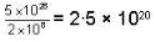donor atoms per met3. This density of doner
Nd = 2-5 x 1020 per m3
We know that Fermi level will coincides with the edge of conduction band if Nd = Nc, where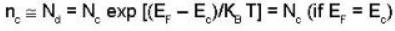with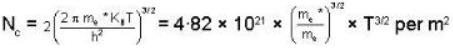But given that effective mass of the electron is equal to its true mass, i.e.,
me* = me
so that
NC = 4.82 x 1021 x T3/2
Putting it equal to Nd, we get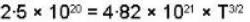or
T = 0.14 K

*Multiple options can be correct
QUESTION: 20

For the circuit shown below:-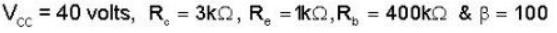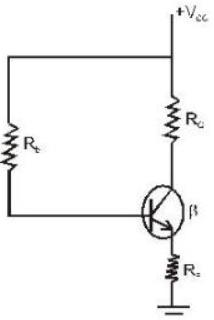Which of the following statement(s) is/are correct?

Solution: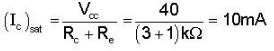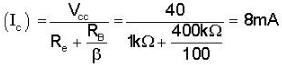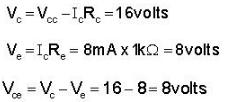*Answer can only contain numeric values
QUESTION: 21

If a transistor has again of 20 dB, then the ratio of output to input power is_____.

Solution: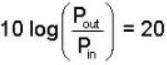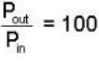*Answer can only contain numeric values
QUESTION: 22

An CE transistor amplifier has a collector current of 1.0mA when its base current is 25μA at room temp. What is the value of its input resistance (in ohms)?

Solution: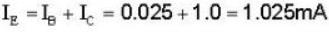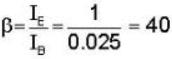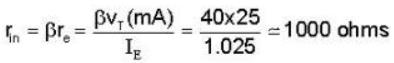*Answer can only contain numeric values
QUESTION: 23

A transistor having α = 0.99 & VBE = 0.7V is used in the circuit shown figure. What is the value of collector current (in mA)?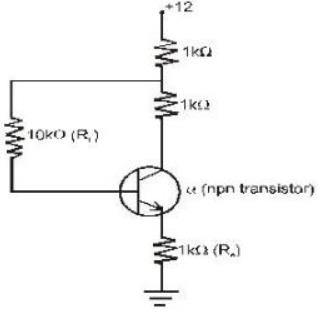Solution:

Taking KVL for the base circuit;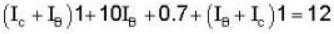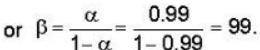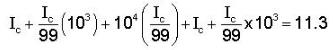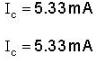*Answer can only contain numeric values
QUESTION: 24

For the circuit,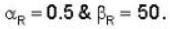If the saturation current is 10-16A . Find the value of base-emitter voltage (in V).

Solution: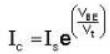Here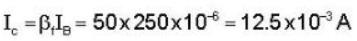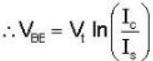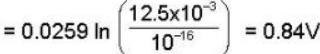*Answer can only contain numeric values
QUESTION: 25

If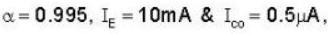then calculate the value of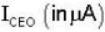?

Solution: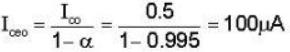*Answer can only contain numeric values
QUESTION: 26

A String under a tension of 129.6 N produces 10 beats per sec when is vibrated along with a tuning frok. when tension in the string is increased to 160N, it sounds in unison with the same tuning frok. calculate the fundamental frequency of a tuning frok? (in Hz)

Solution:

As here the tension in the wire is changed , so its fundamental frequency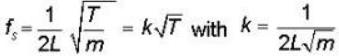Now with increase in tension,
(K√T) will increase & beats & decreasing to zero when
T = 160N (as union means frequencies are equal)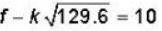...(i)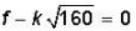...(ii)
Substituting the value of ‘k’ from second equation in first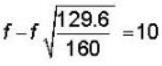f - 0.9 f = 10
f = 100Hz

*Answer can only contain numeric values
QUESTION: 27

A column of air at 51oC & a tuning frok produce 4 beats per sec. when sounded togther. As the temperature of the air column is decreased, the number of beats sec tends to decrease & when the temperature is 16oc , the two produce 1 beat per sec find the frequency of the tuning frok (in Hz)

Solution:

As for a given column of air f α v & V α √T
So ;  f = k√T
If the frequency of the frok is ‘f'
f - k √T = 4...(i)
OR
k√T - f = 4 ...(ii)
But as with decrease in temperature beats decrease, first choice is not permissible ;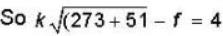i.e (f+4) = 18k ...(iii)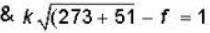i.e (f+1) = 17 k ...(iv)
So dividing eq(3) & (4) we get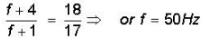*Answer can only contain numeric values
QUESTION: 28

Calculate the thickness of a soap bubble film (refractive index μ =1.3) that will result in constructive interference in the reflected light if the film is illuminated with light whose wavelength in free space is 5000 A0 (Thickness (+) is in Ao)?

Solution:

The condition for constructive interference in the reflected light or fro the brightness of soap bubble film is:-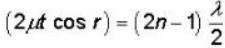When, n = 0 ,1 ,2 ,3 ..............
If the light is incident normally on the film, then r = 0
& cos r = 1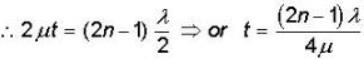putting the given values , we get:-
for least thickness of the film (n=1)
∴ least thickness of the film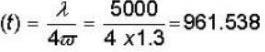t = 961.53 A0

*Answer can only contain numeric values
QUESTION: 29

In the given circuit Vcc = 8V and β = 80 for the n-p-n transistor.The collector voltage V(in volts) is_____.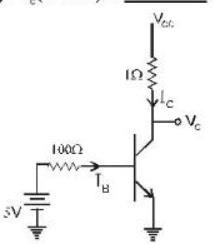Solution:

Applying KVL
5 — 100IB - 0.7 = 0
4.3/100 = IB
I= 43mA
Ic = 80X 43mA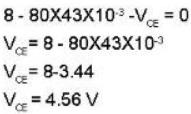*Answer can only contain numeric values
QUESTION: 30

The maximum value of RL so that zener diode is 'ON ' is______(in kΩ).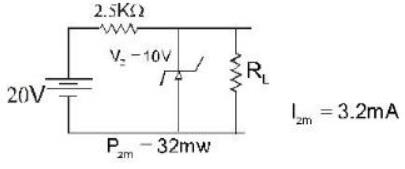Solution: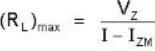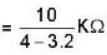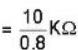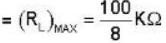= 12.5KΩ